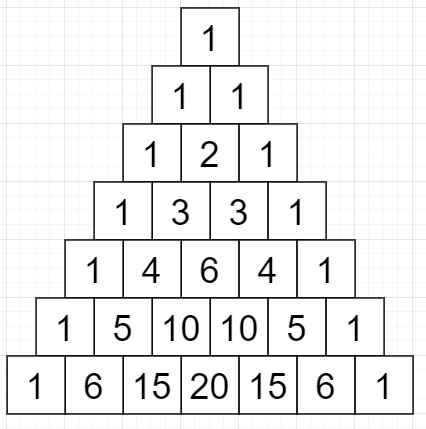# Program to find the nth row of Pascal's Triangle in Python

Suppose we have a number n, we have to find the nth (0-indexed) row of Pascal's triangle. As we know the Pascal's triangle can be created as follows −

• In the top row, there is an array of 1.
• Subsequent row is made by adding the number above and to the left with the number above and to the right.

So few rows are as follows −So, if the input is like 4, then the output will be [1, 4, 6, 4, 1]

To solve this, we will follow these steps −

• if n is same as 0, then
• return 
• if n is same as 1, then
• return [1,1]
• ls:= a list with [1,1], temp:= a list with [1,1]
• for i in range 2 to n+1, do
• ls:= temp
• temp:= a list with one value = 1
• for i in range 0 to size of ls -1, do
• merge ls[i],ls[i+1] and insert at the end of temp
• insert 1 at the end of temp
• return temp

Let us see the following implementation to get better understanding −

## Example

Live Demo

class Solution:
def solve(self, n):
if n==0:
return 
if n==1:
return [1,1]
ls=[1,1]
temp=[1,1]
for i in range(2,n+1):
ls=temp
temp=
for i in range(len(ls)-1):
temp.append(ls[i]+ls[i+1])
temp.append(1)
return temp
ob = Solution()
print(ob.solve(4))

## Input

4

## Output

[1, 4, 6, 4, 1]